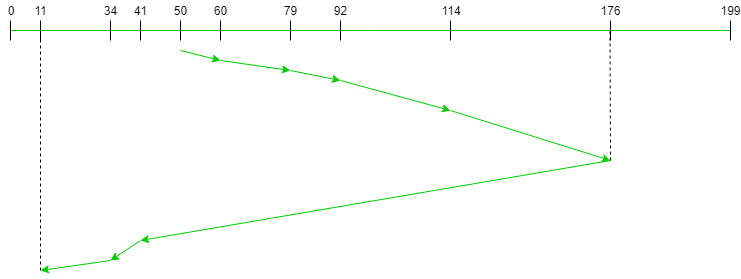# LOOK Disk Scheduling Algorithm

Prerequisite: Disk Scheduling Algorithms

Given an array of disk track numbers and initial head position, our task is to find the total number of seek operations done to access all the requested tracks if LOOK disk scheduling algorithm is used. Also, write a program to find the seek sequence using LOOK disk scheduling algorithm.

LOOK Disk Scheduling Algorithm:
LOOK is the advanced version of SCAN (elevator) disk scheduling algorithm which gives slightly better seek time than any other algorithm in the hierarchy (FCFS->SRTF->SCAN->C-SCAN->LOOK). The LOOK algorithm services request similarly as SCAN algorithm meanwhile it also “looks” ahead as if there are more tracks that are needed to be serviced in the same direction. If there are no pending requests in the moving direction the head reverses the direction and start servicing requests in the opposite direction.

The main reason behind the better performance of LOOK algorithm in comparison to SCAN is because in this algorithm the head is not allowed to move till the end of the disk.

Algorithm:

1. Let Request array represents an array storing indexes of tracks that have been requested in ascending order of their time of arrival. ‘head’ is the position of disk head.
2. The intial direction in which head is moving is given and it services in the same direction.
3. The head services all the requests one by one in the direction head is moving.
4. The head continues to move in the same direction untill all the request in this direction are not finished.
5. While moving in this direction calculate the absolute distance of the track from the head.
6. Increment the total seek count with this distance.
7. Currently serviced track position now becomes the new head position.
8. Go to step 5 until we reach at last request in this direction.
9. If we reach where no requests are needed to be serviced in this direction reverse the direction and go to step 3 until all tracks in request array have not been serviced.

Examples:

```Input:
Request sequence = {176, 79, 34, 60, 92, 11, 41, 114}
Direction = right (We are moving from left to right)

Output:
Total number of seek operations = 291
Seek Sequence is
60
79
92
114
176
41
34
11 ```

The following chart shows the sequence in which requested tracks are serviced using LOOK.Therefore, the total seek count is calculated as:

```= (60-50)+(79-60)+(92-79)
+(114-92)+(176-114)
+(176-41)+(41-34)+(34-11)```

Implementation:
Implementation of LOOK algorithm is given below.

Note: The distance variable is used to store the absolute distance between the head and current track position. disk_size is the size of the disk. Vectors left and right stores all the request tracks on the left-hand side and the right-hand side of the initial head position respectively.

 `#include ` `using namespace std; ` ` `  `// Code by Vikram Chaurasia ` ` `  `// C++ program to demonstrate ` `// SCAN Disk Scheduling algorithm ` ` `  `int size = 8; ` `int disk_size = 200; ` ` `  `void LOOK(int arr[], int head, string direction) ` `{ ` `    ``int seek_count = 0; ` `    ``int distance, cur_track; ` `    ``vector left, right; ` `    ``vector seek_sequence; ` ` `  `    ``// appending values which are ` `    ``// currently at left and right ` `    ``// direction from the head. ` `    ``for (int i = 0; i < size; i++) { ` `        ``if (arr[i] < head) ` `            ``left.push_back(arr[i]); ` `        ``if (arr[i] > head) ` `            ``right.push_back(arr[i]); ` `    ``} ` ` `  `    ``// sorting left and right vectors ` `    ``// for servicing tracks in the ` `    ``// correct sequence. ` `    ``std::sort(left.begin(), left.end()); ` `    ``std::sort(right.begin(), right.end()); ` ` `  `    ``// run the while loop two times. ` `    ``// one by one scanning right ` `    ``// and left side of the head ` `    ``int run = 2; ` `    ``while (run--) { ` `        ``if (direction == "left") { ` `            ``for (int i = left.size() - 1; i >= 0; i--) { ` `                ``cur_track = left[i]; ` ` `  `                ``// appending current track to seek sequence ` `                ``seek_sequence.push_back(cur_track); ` ` `  `                ``// calculate absolute distance ` `                ``distance = abs(cur_track - head); ` ` `  `                ``// increase the total count ` `                ``seek_count += distance; ` ` `  `                ``// accessed track is now the new head ` `                ``head = cur_track; ` `            ``} ` `            ``// reversing the direction ` `            ``direction = "right"; ` `        ``} ` `        ``else if (direction == "right") { ` `            ``for (int i = 0; i < right.size(); i++) { ` `                ``cur_track = right[i]; ` `                ``// appending current track to seek sequence ` `                ``seek_sequence.push_back(cur_track); ` ` `  `                ``// calculate absolute distance ` `                ``distance = abs(cur_track - head); ` ` `  `                ``// increase the total count ` `                ``seek_count += distance; ` ` `  `                ``// accessed track is now new head ` `                ``head = cur_track; ` `            ``} ` `            ``// reversing the direction ` `            ``direction = "left"; ` `        ``} ` `    ``} ` ` `  `    ``cout << "Total number of seek operations = " ` `         ``<< seek_count << endl; ` ` `  `    ``cout << "Seek Sequence is" << endl; ` ` `  `    ``for (int i = 0; i < seek_sequence.size(); i++) { ` `        ``cout << seek_sequence[i] << endl; ` `    ``} ` `} ` ` `  `// Driver code ` `int main() ` `{ ` ` `  `    ``// request array ` `    ``int arr[size] = { 176, 79, 34, 60, ` `                      ``92, 11, 41, 114 }; ` `    ``int head = 50; ` `    ``string direction = "right"; ` ` `  `    ``cout << "Initial position of head: " ` `         ``<< head << endl; ` ` `  `    ``LOOK(arr, head, direction); ` ` `  `    ``return 0; ` `} `

Output:

```Initial position of head: 50
Total number of seek operations = 291
Seek Sequence is
60
79
92
114
176
41
34
11 ```My Personal Notes arrow_drop_upCheck out this Author's contributed articles.

If you like GeeksforGeeks and would like to contribute, you can also write an article using contribute.geeksforgeeks.org or mail your article to contribute@geeksforgeeks.org. See your article appearing on the GeeksforGeeks main page and help other Geeks.

Please Improve this article if you find anything incorrect by clicking on the "Improve Article" button below.